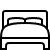## 6 Bedroom House in Gerald Road BOURNEMOUTH

£2,550 PCM
- Fees Apply
Let Agreed6Beds2Baths
• Let
Agreed
• Let
Agreed
• Let
Agreed
• Let
Agreed
• Let
Agreed
• Let
Agreed
• Let
Agreed
• Let
Agreed
• Let
Agreed
• Let
Agreed
• Let
Agreed
• Let
Agreed
• Let
Agreed